# QRectF Class

The QRectF class defines a rectangle in the plane using floating point precision. More...

 Header: #include qmake: QT += core

Note: All functions in this class are reentrant.

## Public Functions

 QRectF() QRectF(const QPointF &topLeft, const QSizeF &size) QRectF(const QPointF &topLeft, const QPointF &bottomRight) QRectF(qreal x, qreal y, qreal width, qreal height) QRectF(const QRect &rectangle) void adjust(qreal dx1, qreal dy1, qreal dx2, qreal dy2) QRectF adjusted(qreal dx1, qreal dy1, qreal dx2, qreal dy2) const qreal bottom() const QPointF bottomLeft() const QPointF bottomRight() const QPointF center() const bool contains(const QPointF &point) const bool contains(const QRectF &rectangle) const bool contains(qreal x, qreal y) const void getCoords(qreal *x1, qreal *y1, qreal *x2, qreal *y2) const void getRect(qreal *x, qreal *y, qreal *width, qreal *height) const qreal height() const QRectF intersected(const QRectF &rectangle) const bool intersects(const QRectF &rectangle) const bool isEmpty() const bool isNull() const bool isValid() const qreal left() const QRectF marginsAdded(const QMarginsF &margins) const QRectF marginsRemoved(const QMarginsF &margins) const void moveBottom(qreal y) void moveBottomLeft(const QPointF &position) void moveBottomRight(const QPointF &position) void moveCenter(const QPointF &position) void moveLeft(qreal x) void moveRight(qreal x) void moveTo(qreal x, qreal y) void moveTo(const QPointF &position) void moveTop(qreal y) void moveTopLeft(const QPointF &position) void moveTopRight(const QPointF &position) QRectF normalized() const qreal right() const void setBottom(qreal y) void setBottomLeft(const QPointF &position) void setBottomRight(const QPointF &position) void setCoords(qreal x1, qreal y1, qreal x2, qreal y2) void setHeight(qreal height) void setLeft(qreal x) void setRect(qreal x, qreal y, qreal width, qreal height) void setRight(qreal x) void setSize(const QSizeF &size) void setTop(qreal y) void setTopLeft(const QPointF &position) void setTopRight(const QPointF &position) void setWidth(qreal width) void setX(qreal x) void setY(qreal y) QSizeF size() const QRect toAlignedRect() const CGRect toCGRect() const QRect toRect() const qreal top() const QPointF topLeft() const QPointF topRight() const void translate(qreal dx, qreal dy) void translate(const QPointF &offset) QRectF translated(qreal dx, qreal dy) const QRectF translated(const QPointF &offset) const QRectF transposed() const QRectF united(const QRectF &rectangle) const qreal width() const qreal x() const qreal y() const QRectF operator&(const QRectF &rectangle) const QRectF & operator&=(const QRectF &rectangle) QRectF & operator+=(const QMarginsF &margins) QRectF & operator-=(const QMarginsF &margins) QRectF operator|(const QRectF &rectangle) const QRectF & operator|=(const QRectF &rectangle)

## Static Public Members

 QRectF fromCGRect(CGRect rect)
 bool operator!=(const QRectF &r1, const QRectF &r2) QRectF operator+(const QRectF &lhs, const QMarginsF &rhs) QRectF operator+(const QMarginsF &lhs, const QRectF &rhs) QRectF operator-(const QRectF &lhs, const QMarginsF &rhs) QDataStream & operator<<(QDataStream &stream, const QRectF &rectangle) bool operator==(const QRectF &r1, const QRectF &r2) QDataStream & operator>>(QDataStream &stream, QRectF &rectangle)

## Detailed Description

The QRectF class defines a rectangle in the plane using floating point precision.

A rectangle is normally expressed as a top-left corner and a size. The size (width and height) of a QRectF is always equivalent to the mathematical rectangle that forms the basis for its rendering.

A QRectF can be constructed with a set of left, top, width and height coordinates, or from a QPointF and a QSizeF. The following code creates two identical rectangles.

```QRectF r1(100.0, 200.1, 11.2, 16.3);
QRectF r2(QPointF(100.0, 200.1), QSizeF(11.2, 16.3));```

There is also a third constructor creating a QRectF from a QRect, and a corresponding toRect() function that returns a QRect object based on the values of this rectangle (note that the coordinates in the returned rectangle are rounded to the nearest integer).

The QRectF class provides a collection of functions that return the various rectangle coordinates, and enable manipulation of these. QRectF also provides functions to move the rectangle relative to the various coordinates. In addition there is a moveTo() function that moves the rectangle, leaving its top left corner at the given coordinates. Alternatively, the translate() function moves the rectangle the given offset relative to the current position, and the translated() function returns a translated copy of this rectangle.

The size() function returns the rectange's dimensions as a QSizeF. The dimensions can also be retrieved separately using the width() and height() functions. To manipulate the dimensions use the setSize(), setWidth() or setHeight() functions. Alternatively, the size can be changed by applying either of the functions setting the rectangle coordinates, for example, setBottom() or setRight().

The contains() function tells whether a given point is inside the rectangle or not, and the intersects() function returns `true` if this rectangle intersects with a given rectangle (otherwise false). The QRectF class also provides the intersected() function which returns the intersection rectangle, and the united() function which returns the rectangle that encloses the given rectangle and this: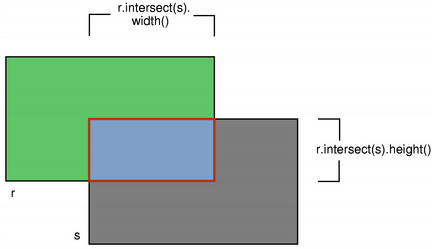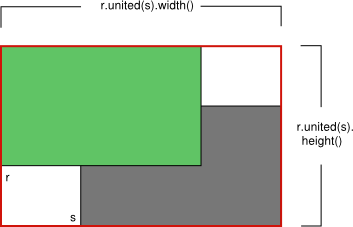intersected() united()

The isEmpty() function returns `true` if the rectangle's width or height is less than, or equal to, 0. Note that an empty rectangle is not valid: The isValid() function returns `true` if both width and height is larger than 0. A null rectangle (isNull() == true) on the other hand, has both width and height set to 0.

Note that due to the way QRect and QRectF are defined, an empty QRectF is defined in essentially the same way as QRect.

Finally, QRectF objects can be streamed as well as compared.

### Rendering

When using an anti-aliased painter, the boundary line of a QRectF will be rendered symmetrically on both sides of the mathematical rectangle's boundary line. But when using an aliased painter (the default) other rules apply.

Then, when rendering with a one pixel wide pen the QRectF's boundary line will be rendered to the right and below the mathematical rectangle's boundary line.

When rendering with a two pixels wide pen the boundary line will be split in the middle by the mathematical rectangle. This will be the case whenever the pen is set to an even number of pixels, while rendering with a pen with an odd number of pixels, the spare pixel will be rendered to the right and below the mathematical rectangle as in the one pixel case.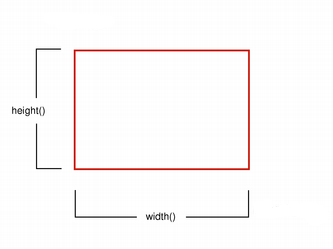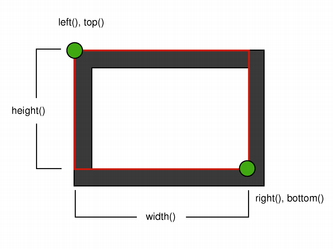Logical representation One pixel wide pen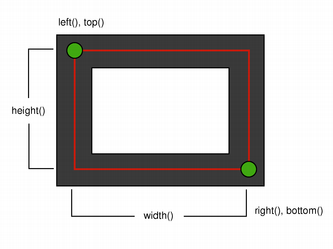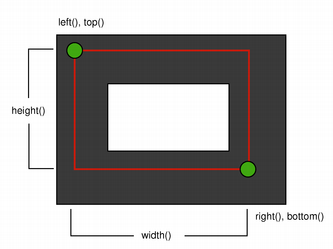Two pixel wide pen Three pixel wide pen

### Coordinates

The QRectF class provides a collection of functions that return the various rectangle coordinates, and enable manipulation of these. QRectF also provides functions to move the rectangle relative to the various coordinates.

For example: the bottom(), setBottom() and moveBottom() functions: bottom() returns the y-coordinate of the rectangle's bottom edge, setBottom() sets the bottom edge of the rectangle to the given y coordinate (it may change the height, but will never change the rectangle's top edge) and moveBottom() moves the entire rectangle vertically, leaving the rectangle's bottom edge at the given y coordinate and its size unchanged.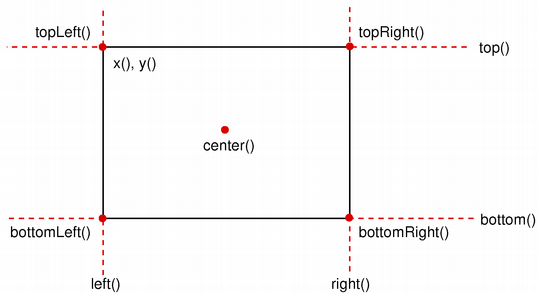It is also possible to add offsets to this rectangle's coordinates using the adjust() function, as well as retrieve a new rectangle based on adjustments of the original one using the adjusted() function. If either of the width and height is negative, use the normalized() function to retrieve a rectangle where the corners are swapped.

In addition, QRectF provides the getCoords() function which extracts the position of the rectangle's top-left and bottom-right corner, and the getRect() function which extracts the rectangle's top-left corner, width and height. Use the setCoords() and setRect() function to manipulate the rectangle's coordinates and dimensions in one go.

## Member Function Documentation

### QRectF::QRectF()

Constructs a null rectangle.

### QRectF::QRectF(const QPointF &topLeft, const QSizeF &size)

Constructs a rectangle with the given topLeft corner and the given size.

### QRectF::QRectF(const QPointF &topLeft, const QPointF &bottomRight)

Constructs a rectangle with the given topLeft and bottomRight corners.

This function was introduced in Qt 4.3.

### QRectF::QRectF(qrealx, qrealy, qrealwidth, qrealheight)

Constructs a rectangle with (x, y) as its top-left corner and the given width and height.

### QRectF::QRectF(const QRect &rectangle)

Constructs a QRectF rectangle from the given QRect rectangle.

### void QRectF::adjust(qrealdx1, qrealdy1, qrealdx2, qrealdy2)

Adds dx1, dy1, dx2 and dy2 respectively to the existing coordinates of the rectangle.

### QRectF QRectF::adjusted(qrealdx1, qrealdy1, qrealdx2, qrealdy2) const

Returns a new rectangle with dx1, dy1, dx2 and dy2 added respectively to the existing coordinates of this rectangle.

### qreal QRectF::bottom() const

Returns the y-coordinate of the rectangle's bottom edge.

### QPointF QRectF::bottomLeft() const

Returns the position of the rectangle's bottom-left corner.

### QPointF QRectF::bottomRight() const

Returns the position of the rectangle's bottom-right corner.

### QPointF QRectF::center() const

Returns the center point of the rectangle.

### bool QRectF::contains(const QPointF &point) const

Returns `true` if the given point is inside or on the edge of the rectangle; otherwise returns `false`.

### bool QRectF::contains(const QRectF &rectangle) const

Returns `true` if the given rectangle is inside this rectangle; otherwise returns `false`.

### bool QRectF::contains(qrealx, qrealy) const

Returns `true` if the point (x, y) is inside or on the edge of the rectangle; otherwise returns `false`.

### `[static]`QRectF QRectF::fromCGRect(CGRectrect)

Creates a QRectF from CGRect rect.

This function was introduced in Qt 5.8.

### void QRectF::getCoords(qreal *x1, qreal *y1, qreal *x2, qreal *y2) const

Extracts the position of the rectangle's top-left corner to *x1 and *y1, and the position of the bottom-right corner to *x2 and *y2.

### void QRectF::getRect(qreal *x, qreal *y, qreal *width, qreal *height) const

Extracts the position of the rectangle's top-left corner to *x and *y, and its dimensions to *width and *height.

### qreal QRectF::height() const

Returns the height of the rectangle.

### QRectF QRectF::intersected(const QRectF &rectangle) const

Returns the intersection of this rectangle and the given rectangle. Note that `r.intersected(s)` is equivalent to `r & s`.This function was introduced in Qt 4.2.

### bool QRectF::intersects(const QRectF &rectangle) const

Returns `true` if this rectangle intersects with the given rectangle (i.e. there is a non-empty area of overlap between them), otherwise returns `false`.

The intersection rectangle can be retrieved using the intersected() function.

### bool QRectF::isEmpty() const

Returns `true` if the rectangle is empty, otherwise returns `false`.

An empty rectangle has width() <= 0 or height() <= 0. An empty rectangle is not valid (i.e., isEmpty() == !isValid()).

Use the normalized() function to retrieve a rectangle where the corners are swapped.

### bool QRectF::isNull() const

Returns `true` if the rectangle is a null rectangle, otherwise returns `false`.

A null rectangle has both the width and the height set to 0. A null rectangle is also empty, and hence not valid.

### bool QRectF::isValid() const

Returns `true` if the rectangle is valid, otherwise returns `false`.

A valid rectangle has a width() > 0 and height() > 0. Note that non-trivial operations like intersections are not defined for invalid rectangles. A valid rectangle is not empty (i.e., isValid() == !isEmpty()).

### qreal QRectF::left() const

Returns the x-coordinate of the rectangle's left edge. Equivalent to x().

### QRectF QRectF::marginsAdded(const QMarginsF &margins) const

Returns a rectangle grown by the margins.

This function was introduced in Qt 5.3.

### QRectF QRectF::marginsRemoved(const QMarginsF &margins) const

Removes the margins from the rectangle, shrinking it.

This function was introduced in Qt 5.3.

### void QRectF::moveBottom(qrealy)

Moves the rectangle vertically, leaving the rectangle's bottom edge at the given y coordinate. The rectangle's size is unchanged.

### void QRectF::moveBottomLeft(const QPointF &position)

Moves the rectangle, leaving the bottom-left corner at the given position. The rectangle's size is unchanged.

### void QRectF::moveBottomRight(const QPointF &position)

Moves the rectangle, leaving the bottom-right corner at the given position. The rectangle's size is unchanged.

### void QRectF::moveCenter(const QPointF &position)

Moves the rectangle, leaving the center point at the given position. The rectangle's size is unchanged.

### void QRectF::moveLeft(qrealx)

Moves the rectangle horizontally, leaving the rectangle's left edge at the given x coordinate. The rectangle's size is unchanged.

### void QRectF::moveRight(qrealx)

Moves the rectangle horizontally, leaving the rectangle's right edge at the given x coordinate. The rectangle's size is unchanged.

### void QRectF::moveTo(qrealx, qrealy)

Moves the rectangle, leaving the top-left corner at the given position (x, y). The rectangle's size is unchanged.

### void QRectF::moveTo(const QPointF &position)

Moves the rectangle, leaving the top-left corner at the given position.

### void QRectF::moveTop(qrealy)

Moves the rectangle vertically, leaving the rectangle's top line at the given y coordinate. The rectangle's size is unchanged.

### void QRectF::moveTopLeft(const QPointF &position)

Moves the rectangle, leaving the top-left corner at the given position. The rectangle's size is unchanged.

### void QRectF::moveTopRight(const QPointF &position)

Moves the rectangle, leaving the top-right corner at the given position. The rectangle's size is unchanged.

### QRectF QRectF::normalized() const

Returns a normalized rectangle; i.e., a rectangle that has a non-negative width and height.

If width() < 0 the function swaps the left and right corners, and it swaps the top and bottom corners if height() < 0.

Returns the x-coordinate of the rectangle's right edge.

### void QRectF::setBottom(qrealy)

Sets the bottom edge of the rectangle to the given y coordinate. May change the height, but will never change the top edge of the rectangle.

### void QRectF::setBottomLeft(const QPointF &position)

Set the bottom-left corner of the rectangle to the given position. May change the size, but will never change the top-right corner of the rectangle.

### void QRectF::setBottomRight(const QPointF &position)

Set the bottom-right corner of the rectangle to the given position. May change the size, but will never change the top-left corner of the rectangle.

### void QRectF::setCoords(qrealx1, qrealy1, qrealx2, qrealy2)

Sets the coordinates of the rectangle's top-left corner to (x1, y1), and the coordinates of its bottom-right corner to (x2, y2).

### void QRectF::setHeight(qrealheight)

Sets the height of the rectangle to the given height. The bottom edge is changed, but not the top one.

### void QRectF::setLeft(qrealx)

Sets the left edge of the rectangle to the given x coordinate. May change the width, but will never change the right edge of the rectangle.

Equivalent to setX().

### void QRectF::setRect(qrealx, qrealy, qrealwidth, qrealheight)

Sets the coordinates of the rectangle's top-left corner to (x, y), and its size to the given width and height.

### void QRectF::setRight(qrealx)

Sets the right edge of the rectangle to the given x coordinate. May change the width, but will never change the left edge of the rectangle.

### void QRectF::setSize(const QSizeF &size)

Sets the size of the rectangle to the given size. The top-left corner is not moved.

### void QRectF::setTop(qrealy)

Sets the top edge of the rectangle to the given y coordinate. May change the height, but will never change the bottom edge of the rectangle.

Equivalent to setY().

### void QRectF::setTopLeft(const QPointF &position)

Set the top-left corner of the rectangle to the given position. May change the size, but will never change the bottom-right corner of the rectangle.

### void QRectF::setTopRight(const QPointF &position)

Set the top-right corner of the rectangle to the given position. May change the size, but will never change the bottom-left corner of the rectangle.

### void QRectF::setWidth(qrealwidth)

Sets the width of the rectangle to the given width. The right edge is changed, but not the left one.

### void QRectF::setX(qrealx)

Sets the left edge of the rectangle to the given x coordinate. May change the width, but will never change the right edge of the rectangle.

Equivalent to setLeft().

### void QRectF::setY(qrealy)

Sets the top edge of the rectangle to the given y coordinate. May change the height, but will never change the bottom edge of the rectangle.

Equivalent to setTop().

### QSizeF QRectF::size() const

Returns the size of the rectangle.

### QRect QRectF::toAlignedRect() const

Returns a QRect based on the values of this rectangle that is the smallest possible integer rectangle that completely contains this rectangle.

This function was introduced in Qt 4.3.

### CGRect QRectF::toCGRect() const

Creates a CGRect from a QRectF.

This function was introduced in Qt 5.8.

### QRect QRectF::toRect() const

Returns a QRect based on the values of this rectangle. Note that the coordinates in the returned rectangle are rounded to the nearest integer.

### qreal QRectF::top() const

Returns the y-coordinate of the rectangle's top edge. Equivalent to y().

### QPointF QRectF::topLeft() const

Returns the position of the rectangle's top-left corner.

### QPointF QRectF::topRight() const

Returns the position of the rectangle's top-right corner.

### void QRectF::translate(qrealdx, qrealdy)

Moves the rectangle dx along the x-axis and dy along the y-axis, relative to the current position. Positive values move the rectangle to the right and downwards.

### void QRectF::translate(const QPointF &offset)

Moves the rectangle offset.x() along the x axis and offset.y() along the y axis, relative to the current position.

### QRectF QRectF::translated(qrealdx, qrealdy) const

Returns a copy of the rectangle that is translated dx along the x axis and dy along the y axis, relative to the current position. Positive values move the rectangle to the right and down.

### QRectF QRectF::translated(const QPointF &offset) const

Returns a copy of the rectangle that is translated offset.x() along the x axis and offset.y() along the y axis, relative to the current position.

### QRectF QRectF::transposed() const

Returns a copy of the rectangle that has its width and height exchanged:

```QRectF r = {1.5, 5.1, 4.2, 2.4};
r = r.transposed(); // r == {1.5, 5.1, 2.4, 4.2}```

This function was introduced in Qt 5.7.

### QRectF QRectF::united(const QRectF &rectangle) const

Returns the bounding rectangle of this rectangle and the given rectangle.This function was introduced in Qt 4.2.

### qreal QRectF::width() const

Returns the width of the rectangle.

### qreal QRectF::x() const

Returns the x-coordinate of the rectangle's left edge. Equivalent to left().

### qreal QRectF::y() const

Returns the y-coordinate of the rectangle's top edge. Equivalent to top().

### QRectF QRectF::operator&(const QRectF &rectangle) const

Returns the intersection of this rectangle and the given rectangle. Returns an empty rectangle if there is no intersection.

### QRectF &QRectF::operator&=(const QRectF &rectangle)

Intersects this rectangle with the given rectangle.

### QRectF &QRectF::operator+=(const QMarginsF &margins)

Adds the margins to the rectangle, growing it.

This function was introduced in Qt 5.3.

### QRectF &QRectF::operator-=(const QMarginsF &margins)

Returns a rectangle shrunk by the margins.

This function was introduced in Qt 5.3.

### QRectF QRectF::operator|(const QRectF &rectangle) const

Returns the bounding rectangle of this rectangle and the given rectangle.

### QRectF &QRectF::operator|=(const QRectF &rectangle)

Unites this rectangle with the given rectangle.

## Related Non-Members

### booloperator!=(const QRectF &r1, const QRectF &r2)

Returns `true` if the rectangles r1 and r2 are different, otherwise returns `false`.

### QRectFoperator+(const QRectF &lhs, const QMarginsF &rhs)

Returns the lhs rectangle grown by the rhs margins.

This function was introduced in Qt 5.3.

### QRectFoperator+(const QMarginsF &lhs, const QRectF &rhs)

Returns the lhs rectangle grown by the rhs margins.

This function was introduced in Qt 5.3.

### QRectFoperator-(const QRectF &lhs, const QMarginsF &rhs)

Returns the lhs rectangle grown by the rhs margins.

This function was introduced in Qt 5.3.

### QDataStream &operator<<(QDataStream &stream, const QRectF &rectangle)

Writes the rectangle to the stream, and returns a reference to the stream.

Returns `true` if the rectangles r1 and r2 are equal, otherwise returns `false`.